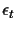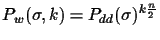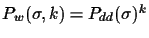## Dynamic Programming Approach

The dynamic programming version of our algorithm is very simple: instead of connecting the two leaves with the smallest error, the best k connections are chosen (where k is a user specified parameter). At the end, the tree with the overall smallest erroris chosen. If the number of trees at the end is t, then the probability get an edge x wrong is much lower: in order to get a wrong connection, all k connections have to be wrong:in the best case, andin the worst case.
To show the difference we build the same table again, for k=3:If we would simply keep all k trees at each step, the number of resulting would grow exponentially. In the worst case, we would get 2n-3 trees, as this is the number of possible different trees that can be built given a circular order. Note that this can only happen in the worst case, if the tree is completely unbalanced (if the tree depth is almost n). In real cases, many trees will end up being the same during the construction process. I.e. if we first connect leaves 1, 2 and then 3, 4 or first 3, 4 and then 1, 2 does not matter. The number of different trees at the end is seldom more than 100 when performing experiments. To identify equal trees during the dynamic programming construction process, a unique number is created for each tree. The number is added to a hash table. Any new tree is compared to the hash table and only kept if it is not already in the list.